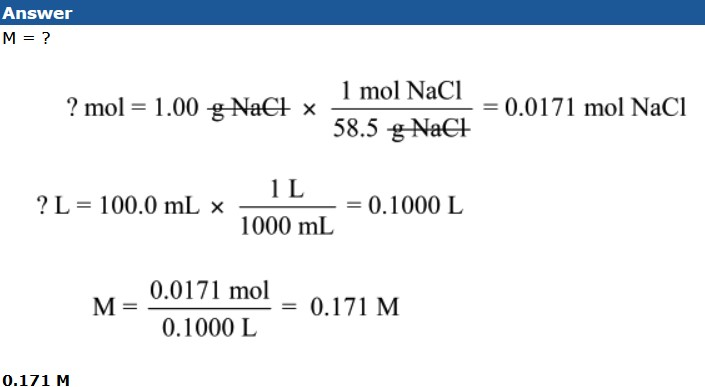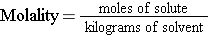# Molarity Problems 126

You try these problems:

Click on Step 1, Step 2, Step 3, Solution, and Answer as applicable to see the solutions and explanations.

Which solution is more concentrated? Solution “A” contains 50.0 g of CaCO3 in 500.0 mL of solution. Solution “B” contains 6.0 moles of H2SO4 in 4.0 L of solution.

Solution A

M = ?
? mol CaCO3 = 50.0 g CaCO3 x (1 mol CaCO3/100.0 g CaCO3) = 0.500 mol CaCO3
? L b= 500.0 mL x (1 L/1000 mL) = 0.500 L
M = 0.500 mol/0.500 L = 1.00 M
M = 1.00 M

Solution B

M = ?
mol = 6.0 mol
L = 4.0 L
M = 6.0 mol/4.0 L = 1.5 M
M = 1.5 M

Solution B is more concentrated, 1.5 M vs. 1.0 M.

How many liters of solution can be produced from 2.5 moles of solute if a 2.0 M solution is needed?

Step 1

M = 2.0 M
mol = 2.5 mol
L = ?

Step 2

Molarity = moles of solute/liters of solution
Multiply both sides by liters of solution, then divide both sides by molarity.
L = mol/M

Step 3

L = mol/M
L = 2.5 mol/2.0 M
L = 1.25 L = 1.3 L

What would be the concentration of a solution formed when 1.00 g of NaCl are dissolved in water to make 100.0 mL of solution?Things to remember when working molarity problems:

• Volume must be in liters.
• An uppercase M is used to denote molarity.
• Use the molar mass of the substance (derived from the periodic table) to determine the moles if grams are given as the starting information.

## Molality

Molality (yes, that’s molality, not a typo!) is another unit of solution concentration. It is defined as:

Molality = moles of solute/kilograms of solventIt is abbreviated m, lowercase m. It is important to differentiate this, because Molarity is M. Other important things to notice about molality are that the denominator is simply kilograms of solvent. Molarity is calculated with liters of solution. That is, the volume of the entire solution (solute + solvent) is used. Molality is calculated with only kilograms of solvent. The mass or volume of the total solution are not useful. Using molality to calculate the concentration of solutions is not used nearly as often as molarity. Because of this, we will not spend much time reviewing molality problems, but we will review one.

Calculate the molality when 45.0 grams of MgCl2 is dissolved in 400.0 g of solvent.

Step 1:

Identify each piece of information given in the problem and identify what the problem is asking for.

m = ?Step 2:

Rearrange the molality equation to solve it for the variable that the question is asking for.Step 3:

Plug in your data from step 1 into the rearranged equation from step 2.

m = 0.473 mol / 0.4000 kg

m = 1.18 m

source